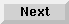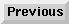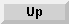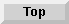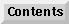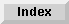4 Data Variables and Data handling

## Numeric Variables

### Real Variable Numeric Ranges

The range of numeric values which can be entered or stored lies between -9.99999999999 x 10125 through -1 x 10-130, 0, and 1 x 10-130 through 9.99999999999 x 10125. The range of intermediate calculations is the same as above, but 16 digits.

### Integer Variable Numeric Range

Integer and dinteger variables hold values between -231 to +231-1. All intermediate calculations are made using the full precision above.

### Numeric Precisions

The precision of a number is a function of its size. The greater the number of digits, the greater the accuracy that the variable is able to record. Of course, the greater the number of digits, the larger the variable and the more storage space it needs. To give you the maximum choice between space and accuracy, Eloquence numeric variables can be stored in any of three forms--INTEGER, SHORT, or REAL (full) precision. The forms used in a program affect the speed of execution, the precision of the results, and the amount of space needed to store the values.

If you do not specify the form, it defaults to REAL (full) precision. Real precision variables are allotted twelve significant digits of precision. They are the most accurate form of holding numeric data but take up the most space.

The following list describes the differing characteristics of the various forms of Eloquence numeric variables. Use the form most appropriate for your own application. Once you have decided, use the declaration statements to specify the format in each program.

• REAL precision variables hold whole or fractional numbers. They are represented internally with a mantissa of 12 significant digits and an exponent in the range from -130 through 125. Although real values offer much greater precision than short values, they occupy twice as much storage space. Note that short values are included in the range of real values. This form is the default. All other numeric variable forms must be explicitly defined.

• SHORT precision variables also hold whole or fractional numbers. They are represented internally with a mantissa of six significant digits and an exponent in the range from -130 through 125.

• INTEGER precision variables hold whole numbers only (no fractional part). Integer-precision numbers range from -231 to +231-1. They are held in binary 2's complement form, (not exponent and mantissa).

• DINTEGER precision variables hold whole numbers only (no fractional part). Integer-precision numbers range from -231 to +231-1. They are held in binary 2's complement form, (not exponent and mantissa).

All numbers are REAL (full) precision unless otherwise defined using a SHORT, INTEGER or DINTEGER statement as shown later. Twelve digits of precision is the maximum. Numbers entered with more than twelve digits will be truncated. In other words, any digits after the twelfth will be ignored. Note that this removes only the least significant digits. For example, entering 1234.5678912365 as the value of a real variable will store 1234.56789124. The same entry, however, will be rounded to 1234.57 in a short variable or 1235 in an integer variable.

NOTE: Confusion can arise between the maximum size of a numeric variable, and its maximum precision. The maximum size of a numeric variable is the largest number it is able to store and is a function of the size of its exponent. The precision shows the accuracy to which the variable is held and is a function of the mantissa size. Thus a real variable may hold a number as large as 9.0 x 1099, much greater than twelve digits in decimal form, but its accuracy is still that of the mantissa.

NOTE: When an INTEGER is loaded from a program into memory is created via a calculation, it takes up to four bytes of main memory, whereas a DINTEGER takes up the same space on memory as on disk. When the INTEGER is stored it is put into two bytes of physical disk space. The DINTEGER remains 4 bytes long. So, if the INTEGER stored, does in fact take more than two bytes of main memory, it will not fit when stored and error 20 ("integer overflow") occurs. To get around this error either store the number in REAL precision, DINTEGER precision, or decrease the size of the INTEGER causing the problem.

### Numeric Display Formats

Three formats are available for displaying and printing numbers--standard, fixed point, and floating point (scientific notation). Standard format is the default; it is automatically set when the machine is switched on or reset. The display format can be changed to fixed or floating point by using the FIXED and FLOAT statements. The STANDARD statement returns the machine to standard format.

Unless IMAGE and PRINT USING statements are used to precisely control the form of output, all numbers are output with a trailing blank and either a leading blank or a minus sign, (if the number output is negative). For more information concerningIMAGE and PRINT USING see "The PRINT Statement" on page 278.

#### Standard Format

The default standard format is convenient for most displays. It is the form commonly used when writing numbers. Standard format is set at power on, RUN, and SCRATCH A. To reset standard format after a FIXED or FLOAT statement has been executed, execute the STANDARD statement:

STANDARD

In standard format, the most significant twelve digits of a number are output. For example, 9876543210.12345 is output as 9876543210.12. Leading and trailing zeros are suppressed. For example, 000032.100000 is output as 32.1.

Any number whose absolute value lies between 1 and 10 is output in fixed format showing all significant digits. Numbers between -1 and 1 are also output in fixed format if they can be represented precisely in 12 or fewer digits to the right of the decimal point. All other numbers are output in scientific notation. The form is the same as FLOAT 11. see "Floating Point Format" on page 87.

Here are a few examples of standard output:

Number Standard Output
15.0015
.23500.235
.0547^94.38415537301E-12
00987987
10000^61.00000000000E+24

#### Fixed Point Format

Fixed format is similar to standard, except with fixed format you can specify the number of digits to appear to the right of the decimal point. If the number output has fewer digits than that specified, trailing zeros are inserted (leading zeros are still suppressed). If the number output has more digits than specified in the FIXED statement then the number is rounded. The following statement sets fixed point format:

FIXED number of digits

The parameter number of digits is a numeric expression, rounded to an integer, which specifies the number of digits to the right of the decimal point. Its range is from 0 to 12.

NOTE: Even if the number displayed is an integer, fixed notation adds a decimal point and the required number of trailing zeros. Similarly FIXED 12 outputs 12 places of decimals for a SHORT variable. As such a variable only extends to 6 places of decimals. The last 6 places for a short variable in FIXED 12 display are always zeros. The internal accuracy is never affected.

Here are some numbers and their FIXED 4 output form:

Number FIXED 4 Output
1818.0000
-.000006-.0000
-2.75327-2.7533
5.3111E453111.0000
1234567891234.51.23456789123E+12

When fixed point is set and the absolute value number to be output is >= 1E12 or has more than 17 digits, the format temporarily reverts to floating point. For example, in FIXED 12, 100000 is output as 1E+05.

#### Floating Point Format

When working with very large or very small numbers, the floating point format is most convenient. This scientific notation outputs a number as a mantissa in the range >1 and <10, followed by an exponent expressed as a power of 10. Syntax of the FLOAT statement is as follows:

FLOAT number of digits

The parameter number of digits is a numeric expression, rounded to an integer, which specifies the number of digits of precision for the mantissa (the number of digits to the right of the mantissa's decimal point). It ranges from 0 to 11.

A number output in floating point format has the form:

```�d.d  ...  dE�dd
Mantissa   Exponent
```
• If the number is negative, a minus sign will precede the mantissa; if the number is positive or zero, a space precedes it.

• A decimal point follows the first digit, except in FLOAT 0.

• Digits may follow the decimal point; the number of digits after the decimal point is set using the number of digits parameter following the FLOAT statement.

• The character E is followed by a plus sign or minus sign and two digits. This is the exponent, and it represents the power of 10 by which the mantissa should be multiplied in order to express the number in standard format. A negative exponent does not mean a negative number, it means that the number is <1.

Here are some examples of FLOAT 2 format:

Number FLOAT 2 Output
-3.2-3.20E+00
2712.71E+02
26.3772.64E+01
.0000044.00E-06
2.4E782.40E+78

### Rounding

A number is rounded before being displayed or printed if there are more digits to the right of the decimal point than the numeric display format allows. The rounding is performed as follows: The first excess digit on the right is checked. If its value is 5 or greater, the digit to the left is incremented by one; otherwise it is unchanged.

Whenever a number is rounded as a result of a numeric display format, it is only the display which is affected. Internally, the number remains as accurate as the variable holding it will allow. For example, execute the lines below:

```A=1.235
1.235
FIXED 2
A
1.24
FIXED 3
A
1.235
```
The value of A does not alter, only its display.

### Simple Numeric Variables

Any simple numeric variable can be assigned a value using the LET statement. Syntax for this statement is as follows:

[LET] simple variable1 [=simple variable2 . . .]=numeric expression

Notice the keyword LET is optional. For example, each of the following statements assigns the value 12 to X:

```X=12

LET X = 12

X=SQR(144)
```
As another example, the following assigns the value 12 to Y and X:

```X=Y=3*4
```
All variables are set to zero when created or when a program is run. They remain as zero until a value has been assigned to them.

To check the current value of a variable, type in its name, then press RETURN.

The values of simple variables are erased by executing a SCRATCH statement, as shown in page 25 .

### Types and Values

Eloquence is not a strongly typed language. Thus a numeric variable of one type (for example, INTEGER) may be assigned a value from a variable of another (for example, REAL). The value will be converted to the receiving variable's type when transferred. The following example explains further:

```
10 A=1.2345678 ! REAL A implicitly defined as REAL is default
20 SHORT B
30 INTEGER C
40 B=A
50 C=A
60 PRINT "A is ";A;" B is ";B;" C is ";C
70 END
RUN
A is  1.2345678 B is  1.23457 C is  1
```
In this example it is not just the display of the value that is altered. The value is permanently changed in the receiving variable. No error will be indicated in these transfers, but any attempt to assign a string value to a numeric variable (or vice versa) will not be accepted.

### Numeric Arrays

An array is a structured group of data items, all of the same type. Arrays are very useful when manipulating large amounts of associated data, as an array is stored or retrieved as a unit. (When an individual item is required from within an array it may be accessed uniquely.) The individual data items in arrays are called array elements, they have all the properties of simple variables of their type.

An array may have from one to six dimensions. Examples of one and two dimensional arrays are shown below. Arrays of three or more dimensions are not so conveniently represented on paper, but they can be set up and manipulated easily in a program.

 1.5 2.3 3.4 4.7 10.7 0.8 3.5 4.6 2 1.1

 12.95 12.95 11.5 11.5 3.95 3.5 3.5 3.5 0.8 0.8 0.8 0.8

#### Subscripts and Array Size

Every numeric array must be explicitly defined in a variable declarative statement; these are described later in this chapter. There, its size is specified by numbers in parentheses after its name. These numbers are known as subscripts, and their presence tells the Eloquence interpreter to assign dimensions to the array--6 dimensions maximum. Thus a maximum of six numbers, separated by commas, will be accepted. The size of the number tells the interpreter how large the dimension will be. Assigning a size to an array is known as dimensioning an array. The number of elements in an array is the product of the number of elements in each dimension. The above two-dimensional array has one dimension of 3 elements and the other of 4 elements. Their product, 12, gives the number of elements in the array.

An element is accessed by quoting its position in the array. This is done by quoting first the array name and then the subscript(s) which point to the element's position in the array. The number of subscripts which need to be quoted is the same as the number of dimensions. In a two dimensional array there will be two subscripts. It may help, to think of them as coordinates.

Subscripts are integer expressions separated by commas and enclosed in parentheses. (Any non-integer numeric value quoted as a subscript will be rounded to the nearest integer.) If a subscript is outside the range defined for an array (either too large or too small), then ERROR 17 is returned. The range of each subscript is -32767 through 32767, but the size of an array is limited by memory. The size of an array depends on both upper and lower subscript bounds.

For example, an array M dimensioned as M(2,3) is an array with two dimensions having upper bounds of 2 and 3. If the lower bound for each dimension is 0 (the default value), the array has a total of 12 elements. (As the lower bound for each dimension is 0, the total number of elements is "0..2" in the first dimension, and "0..3" in the second. There are thus 3*4 possible combinations of subscript or 12 elements in all.)

Here is a representation of array M:
Columns0 1 2 3
Row 0(0,0)(0,1)(0,2)(0,3)
Row 1(1,0)(1,1)(1,2)(1,3)
Row 2(2,0)(2,1)(2,2)(2,3)

The use of columns and rows is to assist in explaining the concept of arrays, and how an element is accessed. Arrays are not held as grids within the computer, but the principle is the same.

The OPTION BASE statement is used to change the default lower bound, but the lower bound may always be defined for any array dimension by using double subscripts. The array M could also be dimensioned M(-1:1,-2:1). The upper and lower bounds are separated by a colon. Note that the number of elements is still the same; the size of array M has not altered.

The subscripts for array M would now be the following:
Columns-2 -1 0 1
Row -1(-1,-2)(-1,-1)(-1,0)(-1,1)
Row 0(0,-2)(0,-1)(0,0)(0,1)
Row 1(1,-2)(1,-1)(1,0)(1,1)

### Array Elements

Every array element must be of the same type; therefore, you cannot mix REAL and INTEGER elements in one array. As each element in the array is referenced by using subscripts (and can be used like a simple variable), M(1,0) refers to an element in array M which may be assigned a value and used in calculations and other programming operations. For example:

```M(1,0) = 10

A = M(1,0)/7

PRINT M(1,0)
```
Since a subscript is a numeric expression evaluating to an integer, numeric expressions and variables may be used as subscripts. This allows powerful programming constructs:

```30 FOR I = -2 TO 1
50 NEXT I
```
It is thus much easier to loop through the elements of an array, when loading large amounts of data, than to use multiple INPUT statements. The larger the array, the greater the time saved.

All elements of an array can be specified collectively in an input or output operation by using the array identifier. For example:

```PRINT A(*)
```
prints the entire array A. The MAT PRINT statement is also available to print arrays, as shown in page 297 .

The maximum size of an array is specified in a DIM, COM, REAL, SHORT, INTEGER or DINTEGER statement, as shown later in this chapter. If an array is used without being explicitly defined, the default upper bound of the array is set to 10. The lower bound is either 0 or 1, depending on the OPTION BASE setting. To minimize memory waste and make your programs clearer, it is recommended that you define arrays and other variables explicitly at the start of a program.

Eloquence Language Manual - 19 DEC 2002